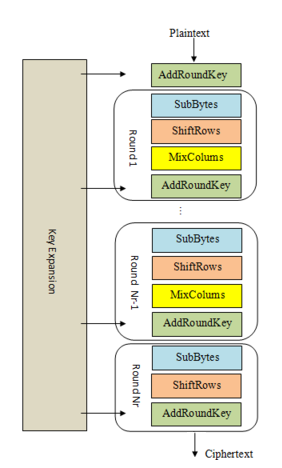## Symmetric Cryptography

• Structure of AES
15 pts · 5966 Solves

To achieve a keyed permutation that is infeasible to invert without the key, AES applies a large number of ad-hoc mixing operations on the input. This is in stark contrast to public-key cryptosystems like RSA, which are based on elegant individual mathematical problems. AES is much less elegant, but it's very fast.

At a high level, AES-128 begins with a "key schedule" and then runs 10 rounds over a state. The starting state is just the plaintext block that we want to encrypt, represented as a 4x4 matrix of bytes. Over the course of the 10 rounds, the state is repeatedly modified by a number of invertible transformations.

Each transformation step has a defined purpose based on theoretical properties of secure ciphers established by Claude Shannon in the 1940s. We'll look closer at each of these in the following challenges.

Here's an overview of the phases of AES encryption:1. KeyExpansion or Key Schedule

From the 128 bit key, 11 separate 128 bit "round keys" are derived: one to be used in each AddRoundKey step.

AddRoundKey - the bytes of the first round key are XOR'd with the bytes of the state.

3. Round - this phase is looped 10 times, for 9 main rounds plus one "final round"

a) SubBytes - each byte of the state is substituted for a different byte according to a lookup table ("S-box").

b) ShiftRows - the last three rows of the state matrix are transposed—shifted over a column or two or three.

c) MixColumns - matrix multiplication is performed on the columns of the state, combining the four bytes in each column. This is skipped in the final round.

d) AddRoundKey - the bytes of the current round key are XOR'd with the bytes of the state.

Included is a `bytes2matrix` function for converting our initial plaintext block into a state matrix. Write a `matrix2bytes` function to turn that matrix back into bytes, and submit the resulting plaintext as the flag.

Challenge files:
- matrix.py

Resources:
- YouTube: AES Rijndael Cipher explained as a Flash animation

You must be logged in to submit your flag.### Level UpYou are now level Current level